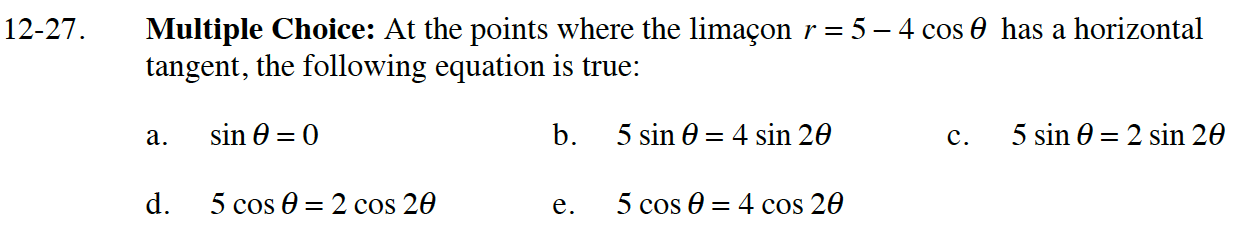### Home > CALC > Chapter 12 > Lesson 12.1.2 > Problem12-27

12-27.This is when dy/ = 0.

$y = r\sin(\theta)=(5-4\cos(\theta))\sin(\theta)$

You will need to use a double angle identity to rewrite your y = equation or your final answer.